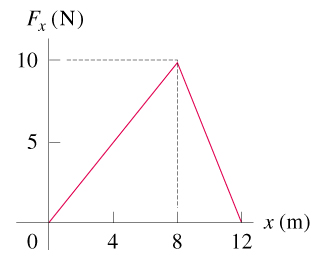# Problem: A child applies a force F parallel to the x-axis to a 8.00-kg sled moving on the frozen surface of a small pond. As the child controls the speed of the sled, the x-component of the force she applies varies with the x-coordinate of the sled as shown in figure . Suppose the sled is initially at rest at x=0. You can ignore friction between the sled and the surface of the pond.You may want to review (Pages 183 - 189).For related problem solving tips and strategies, you may want to view a Video Tutor Solution of Motion on a curved path.(a) Use the work-energy theorem to find the speed of the sled at 5.0 m.(b) Use the work-energy theorem to find the speed of the sled at 11.0 m.

###### FREE Expert Solution

In this problem, we are given a force-distance curve. We are also required to apply the work-energy theorem. In a force-distance graph, we know that work done by the force is equal to the area under the graph.

Work-energy theorem:

$\overline{){{\mathbf{W}}}_{\mathbf{n}\mathbf{e}\mathbf{t}}{\mathbf{=}}{\mathbf{∆}}{\mathbf{K}}{\mathbf{E}}}$

(a)

We'll determine the force at 5m. The graph does not show the position of 5. We'll subdivide the length between 4 and 8 to estimate the location of 5m. We'll also estimate the amount of force, then work out the area under the curve.

83% (444 ratings)###### Problem Details
A child applies a force F parallel to the x-axis to a 8.00-kg sled moving on the frozen surface of a small pond. As the child controls the speed of the sled, the x-component of the force she applies varies with the x-coordinate of the sled as shown in figure. Suppose the sled is initially at rest at x=0. You can ignore friction between the sled and the surface of the pond.

You may want to review (Pages 183 - 189).

For related problem solving tips and strategies, you may want to view a Video Tutor Solution of Motion on a curved path.

(a) Use the work-energy theorem to find the speed of the sled at 5.0 m.

(b) Use the work-energy theorem to find the speed of the sled at 11.0 m.

Frequently Asked Questions

What scientific concept do you need to know in order to solve this problem?

Our tutors have indicated that to solve this problem you will need to apply the Work By Springs concept. You can view video lessons to learn Work By Springs. Or if you need more Work By Springs practice, you can also practice Work By Springs practice problems.

What professor is this problem relevant for?

Based on our data, we think this problem is relevant for Professor Burgasser's class at UCSD.